#### 期刊菜单

An Analysis Method of TT&C Link Based on Boosted Trajectory
DOI: 10.12677/JAST.2021.92007, PDF, HTML, XML, 下载: 213  浏览: 471

Abstract: As an important support for the launch of carrying spacecraft, the tracking telemetering and command (TT&C) of spacecraft launch is of great significance to ensure the safe and fast entry of spacecraft into space. A scheme of real-time antenna direction angle and TT&C link margin calculation for the time of launch of non-associated rocket based on theoretical trajectory and attitude files was proposed. Automatic analysis for any TT&C station of tracking conditions and tracking performance was completed. At the same time, data were generated per second and display was visualized. And simulation example was presented to verify the feasibility and practicability of this method. It can be used to analyze the TT&C link.

1. 引言

2. 坐标系定义及转换关系

2.1. 坐标系定义

1) 地心固连坐标系(e系)

2) 发射坐标系(F系)

3) 发射惯性坐标系(A系)

4) 火箭本体坐标系(b系)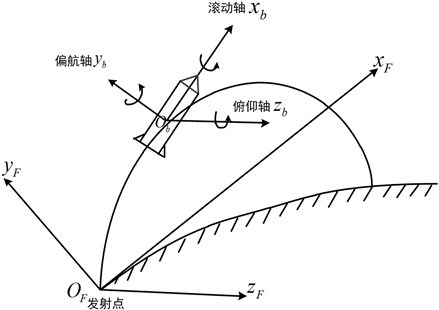Figure 1. The relationship between the rocket body and the launch coordinate system

5) 卫星本体坐标系(s系)

${i}_{a}={\left[\mathrm{cos}{\theta }_{1}\mathrm{cos}{\theta }_{2}\text{\hspace{0.17em}}\text{\hspace{0.17em}}\mathrm{cos}{\theta }_{1}\mathrm{sin}{\theta }_{2}\text{\hspace{0.17em}}\text{\hspace{0.17em}}\mathrm{sin}{\theta }_{1}\right]}^{T}$

1)卫星天线安装俯仰角 ${\theta }_{1}$ ：天线方向与卫星本体系XOY平面上的夹角，指向+Z方向为正，若卫星天线为+Z轴安装，则此值为90度。

2)卫星天线安装方位角 ${\theta }_{2}$ ：天线方向在卫星本体系XOY平面上的投影与X轴的夹角，从卫星本体+Z轴方向向原点看去逆时针方向旋转为正，若卫星天线为+Z轴安装，则此值为0度。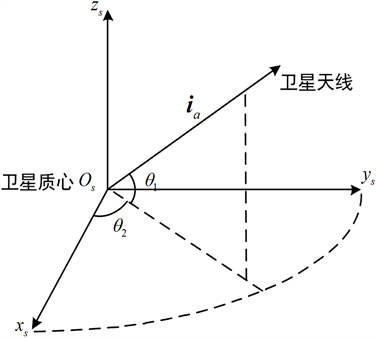Figure 2. Schematic diagram of satellite antenna installation in the satellite body coordinate system

2.2. 坐标转换关系

2.2.1. 地固系到发射坐标系转换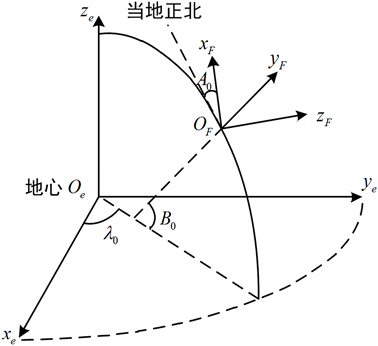Figure 3. The relationship between the Earth fixed and the launch coordinate system

${R}_{e}^{F}={R}_{y}\left[-\left({90}^{\circ }+{A}_{0}\right)\right]{R}_{x}\left[{B}_{0}\right]{R}_{z}\left[-\left({90}^{\circ }-{\lambda }_{0}\right)\right]$ (1)

${R}_{x}\left(\theta \right)=\left[\begin{array}{ccc}1& 0& 0\\ 0& \mathrm{cos}\theta & \mathrm{sin}\theta \\ 0& -\mathrm{sin}\theta & \mathrm{cos}\theta \end{array}\right]$

${R}_{y}\left(\theta \right)=\left[\begin{array}{ccc}\mathrm{cos}\theta & 0& -\mathrm{sin}\theta \\ 0& 1& 0\\ \mathrm{sin}\theta & 0& \mathrm{cos}\theta \end{array}\right]$

${R}_{z}\left(\theta \right)=\left[\begin{array}{ccc}\mathrm{cos}\theta & \mathrm{sin}\theta & 0\\ -\mathrm{sin}\theta & \mathrm{cos}\theta & 0\\ 0& 0& 1\end{array}\right]$

2.2.2. 发射坐标系到发射惯性坐标系转换

${R}_{A}^{F}={I}_{3×3}-\left[{\omega }_{e}^{0}×\right]\mathrm{sin}{\omega }_{e}t+{\left[{\omega }_{e}^{0}×\right]}^{2}\left(1-\mathrm{cos}{\omega }_{e}t\right)$ (2)

$\left[{\omega }_{e}^{0}×\right]=\left[\begin{array}{ccc}0& -{\omega }_{ez}^{0}& {\omega }_{ey}^{0}\\ {\omega }_{ez}^{0}& 0& -{\omega }_{ex}^{0}\\ -{\omega }_{ey}^{0}& {\omega }_{ex}^{0}& 0\end{array}\right]$

$\left\{\begin{array}{l}{\omega }_{ex}^{0}=\mathrm{cos}{B}_{0}\mathrm{cos}{A}_{0}\\ {\omega }_{ey}^{0}=\mathrm{sin}{B}_{0}\\ {\omega }_{ez}^{0}=-\mathrm{cos}{B}_{0}\mathrm{sin}{A}_{0}\end{array}$

${R}_{F}^{A}={\left({R}_{A}^{F}\right)}^{T}$ (3)

2.2.3. 发射惯性坐标系到火箭本体系转换

${R}_{A}^{b}={R}_{x}\left[\gamma \right]{R}_{y}\left[\psi \right]{R}_{z}\left[\varphi \right]$ (4)

2.2.4. 地固系到火箭本体坐标系转换

${R}_{e}^{b}={R}_{A}^{b}{R}_{F}^{A}{R}_{e}^{F}$ (5)

3. 天线测控角计算方法

3.1. 天线测控角定义

3.2. 天线测控角计算

${R}_{sta}=\left[\begin{array}{c}\left(N+{h}_{0}\right)\mathrm{cos}{B}_{0}\mathrm{cos}{\lambda }_{\text{0}}\\ \left(N+{h}_{0}\right)\mathrm{cos}{B}_{0}\mathrm{sin}{\lambda }_{\text{0}}\\ \left[N\left(1-{e}^{2}\right)+{h}_{0}\right]\mathrm{sin}{B}_{0}\end{array}\right]$ (6)

$d{s}^{e}={R}_{sta}-{r}_{roc}$ (7)

$d{s}^{b}={R}_{e}^{b}d{s}^{e}$ (8)

$\alpha =\left\{\begin{array}{l}\mathrm{arctan}2\left(d{s}_{bz},d{s}_{by}\right),\text{\hspace{0.17em}}\text{\hspace{0.17em}}\mathrm{arctan}2\left(d{s}_{bz},d{s}_{by}\right)>0\\ 2\pi +\mathrm{arctan}2\left(d{s}_{bz},d{s}_{by}\right),\text{\hspace{0.17em}}\text{\hspace{0.17em}}\mathrm{arctan}2\left(d{s}_{bz},d{s}_{by}\right)<0\end{array}$ (9)

${i}_{b}={R}_{s}^{b}{i}_{a}$

$\beta =\mathrm{arccos}\left(\frac{{i}_{b}\cdot d{s}^{b}}{|d{s}^{b}|}\right)$ (10)

4. 测控链路电平分析计算

4.1. 卫星接收电平及余量计算

${L}_{s}\left(dB\cdot m\right)=EIR{P}_{1}+{G}_{b}-{L}_{r1}-{L}_{\sum 1}$ (11)

1) $EIR{P}_{1}$ 为地面天线等效各向同性辐射功率，单位为dBW。

2) ${G}_{b}$ 为卫星天线增益，单位为dBi。

3) ${L}_{r1}$ 为上行路径损耗，计算公式为 ${L}_{r1}\left(dB\right)=32.44+20\mathrm{lg}\left({F}_{1}\right)+20\mathrm{lg}\left(R\right)$。其中： ${F}_{1}$ 为卫星上行频率，单位为MHz；R为通信距离，单位为km。

4) ${L}_{\sum 1}$ 为上行其他损耗，单位为dB，包括极化损耗、大气吸收损耗、天线指向误差损耗和馈线损耗等，根据经验取值。

4.2. 地面接收载噪比及余量计算

${L}_{d}\left(dB\cdot Hz\right)=EIR{P}_{2}+{G}_{t}-{L}_{r2}-{L}_{\sum 2}-K$ (12)

1) $EIR{P}_{2}$ 为卫星天线等效各向同性辐射功率，单位为dBW。

2) ${G}_{t}$ 为地面接收G/T值，单位为dB/K。

3) ${L}_{r2}$ 为下行路径损耗，计算公式为 ${L}_{r2}\left(dB\right)=32.44+20\mathrm{lg}\left({F}_{2}\right)+20\mathrm{lg}\left(R\right)$。其中： ${F}_{2}$ 为卫星下行频率，单位为MHz；R为通信距离，单位为km。

4) ${L}_{\sum 2}$ 为下行其他损耗，单位为dB，包括极化损耗、大气吸收损耗、天线指向误差损耗和馈线损耗等，根据经验取值。

5) K为波尔兹曼常数，取定值−228.6 dB/K。

5. 仿真分析

5.1. 设备跟踪卫星天线及动态分析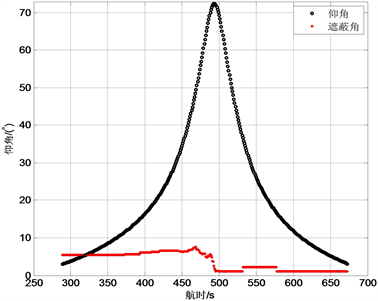Figure 4. The analysis curve of masking visibilityFigure 5. The analysis curve of distance visibility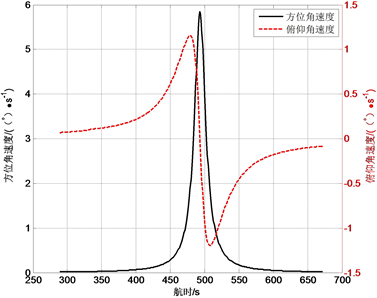Figure 6. Angular velocity dynamic curve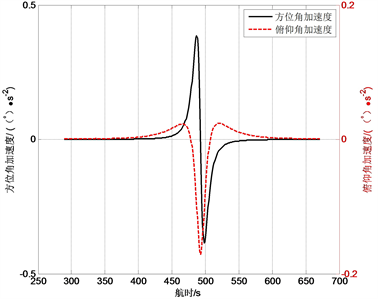Figure 7. Angular acceleration dynamic curve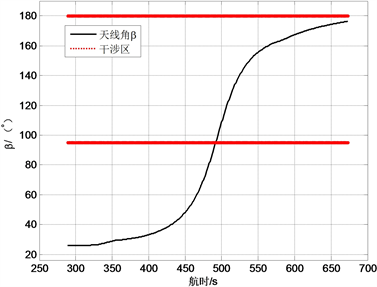Figure 8. Antenna direction angle of the satellite +Z axis

5.2. 设备跟踪卫星测控链路电平分析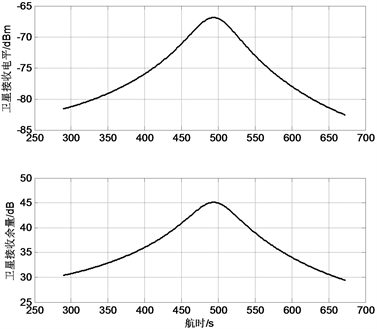Figure 9. Satellite receiving level and margin curve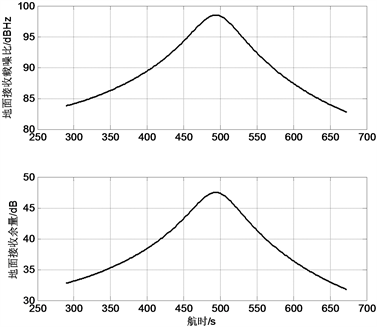Figure 10. Ground receiving carrier-to-noise ratio and margin curve

6. 结论

  郝岩. 航天测控网[M]. 北京: 国防工业出版社, 2004: 31-33.  周国峰, 韩英宏, 陈新民, 等. 基于飞行弹道的数据链链路分析方法[J]. 兵器装备工程学报, 2019, 40(6): 27-31.  余浩章, 吴正容, 王青伟. 箭上天线方向图要求设计方法的改进与优化[J]. 飞行器测控学报, 2010, 29(4): 12-17.  李国军, 霍德聪. 利用STK计算卫星通信链路余量[J]. 空间电子技术, 2012(1): 68-72.  刘蕴才, 张纪生, 黄学德, 等. 导弹卫星测控系统工程[M]. 北京: 国防工业出版社, 1996: 58-65.  贾沛然, 陈克俊, 何力. 远程火箭弹道学[M]. 长沙: 国防科技大学出版社, 1993: 7-16.